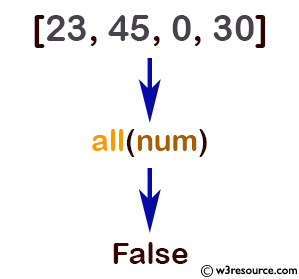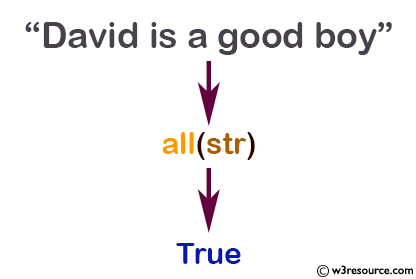# Python: all() function

## all() function

The all() function is used to test whether all elements of an iterable are true or not.

Syntax

```all(iterable)
```Version:

(Python 3)

Parameter:

Name Description Required/ Optional
iterable A container is considered to be iterble, which can return its members sequentially. Required

Return value:

Return True if all elements of the iterable are true.

Python: all() example with Lists

``````# all values true
num = [23, 45, 10, 30]
print(all(num)) #True

# all values false
num = [0, False]
print(all(num)) #False

# one false value
num = [1, 3, 4, 0]
print(all(num)) #False

# one true value
num = [0, False, 5]
print(all(num)) #False

# empty iterable
num = []
print(all(num)) #True
``````

Output:

```False
False
False
False
True
```

Pictorial Presentation:Python: all() example with Dictionaries

``````dict = {0: 'False', 1: 'False'}
print(all(dict)) #False

dict = {1: 'True', 2: 'True'}
print(all(dict)) #True

dict = {1: 'True', False: 0}
print(all(dict)) #False

dict = {}
print(all(dict)) #True

# 0 is False
# '0' is True
dict = {'0': 'True'}
print(all(dict)) #True
``````

Output:

```False
True
False
True
True
```

Python: all() example with Tuple

``````#Test if all items in a tuple are True:
tup = (0, True, False)
x = all(tup)
print(x) #False
tup = (1, True, True)
x = all(tup)
print(x) #True
``````

Output:

```False
True
```

Python: all() example with Set

``````#Test if all items in a set are True:
sss = {0, True, False}
x = all(sss)
print(x) #False
sss = (1, True, True)
x = all(sss)
print(x) #True
``````

Output:

```False
True
False
True
True
```

Example: Python all() function with Strings

``````str = "David is a good boy"
print(all(str)) #True

# 0 is False
# '0' is True
str = '000'
print(all(str)) #True

str = ''
print(all(str)) #True
``````

Output:

```True
True
True
```

Pictorial Presentation:Python Code Editor:

Previous: abs()
Next: any()

Test your Python skills with w3resource's quiz

﻿

## Python: Tips of the Day

How do I check if a list is empty?

For example, if passed the following:

```a = []
if not a:
print("List is empty")
```

Ref: https://bit.ly/2A4JXx9